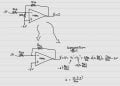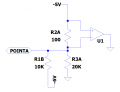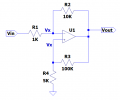# Ideal Opamp current

#### Alec_t

Joined Sep 17, 2013
13,793
Welcome to AAC.
Have you considered what happens when the op-amp output is clamped to ground as shown?

#### Ian0

Joined Aug 7, 2020
8,407
Welcome to AAC.
Have you considered what happens when the op-amp output is clamped to ground as shown?
Nuclear fusion?

#### RoofSheep

Joined Mar 7, 2023
36
Let's assume an ideal opamp in this circuit.
R2A is 100 ohm resistor and my question is .. how does R2A affect this circuit?

Being an ideal opamp and negatively feedback .. V+=V- and therefore no current flows on R2A (zero voltage drop).
Am I wrong?
View attachment 292060
You are right. In the case of an ideal opamp.

#### crutschow

Joined Mar 14, 2008
32,926
You are right. In the case of an ideal opamp.
Not if the output is grounded.

#### Papabravo

Joined Feb 24, 2006
20,399
If both input terminals of the opamp are at the same potential, then no current will flow in resistor R2A. That is just a simple application of Ohm's law. What did you expect?

#### RoofSheep

Joined Mar 7, 2023
36

#### kalemaxon89

Joined Oct 12, 2022
192
If both input terminals of the opamp are at the same potential, then no current will flow in resistor R2A. That is just a simple application of Ohm's law. What did you expect?
The output is 0V ... not ground. I've just realised this detail.
But still I don't think it changes things, so R2A= 100 ohms I don't consider it in the circuit

#### Alec_t

Joined Sep 17, 2013
13,793
But still I don't think it changes things
It definitely does. If the op-amp output is held at 0V it can't do its job to provide negative feedback to minimise the voltage difference between the inverting and non-inverting inputs.

#### LvW

Joined Jun 13, 2013
1,669
The output is 0V ... not ground. I've just realised this detail.
But still I don't think it changes things, so R2A= 100 ohms I don't consider it in the circuit
So - one could/should ask "what is the purpose of this resistor"?
Is there any purpose? The answer is YES.
This resistor has no significant influence on the closed-loop gain.
However, it drastically reduces the feedback factor and, hence, the loop gain because we have to consider R1B||R2A.
In most cases, this resistor R2A is used together with a series capacitor Cs - so that it has no influence on DC feedback.
This loop gain reduction (with a capacitor Cs for higher frequencies only) improves the stability margin.

#### kalemaxon89

Joined Oct 12, 2022
192
So - one could/should ask "what is the purpose of this resistor"?
Is there any purpose? The answer is YES.
This resistor has no significant influence on the closed-loop gain.
However, it drastically reduces the feedback factor and, hence, the loop gain because we have to consider R1B||R2A.
In most cases, this resistor R2A is used together with a series capacitor Cs - so that it has no influence on DC feedback.
This loop gain reduction (with a capacitor Cs for higher frequencies only) improves the stability margin.
It definitely does. If the op-amp output is held at 0V it can't do its job to provide negative feedback to minimise the voltage difference between the inverting and non-inverting inputs.#### DickCappels

Joined Aug 21, 2008
10,068
R2A serves to reduce loop gain, but there is usually a capacitor in series with it so it does not affect offset voltage. Lowering loop gain can stop an opamp circuit from oscillating but it also increased amplification of an opamp’s internal noise.

#### dcbingaman

Joined Jun 30, 2021
978
Sometimes just redrawing the circuit can make certain things obvious, as shown here the op amp is clearly showing it is doing nothing:Because R1B and R3A are at least 100 times greater in value than R2A, we can say the the voltage drop across R2A is negligible and the voltage at point A with respect to ground is just -5V and the current through R3A is then -5V/20K or -250 microamps. The current in R1B then is just (-5V- (-6V))/10K or +100 microamps. The current then through R2A is just the sum of the two or -150 microamps. That is an approximation but close enough for most situations.

Last edited:

#### kalemaxon89

Joined Oct 12, 2022
192
Sometimes just redrawing the circuit can make certain things obvious, as shown here the op amp is clearly showing it is doing nothing:

View attachment 292226
Because R1B and R3A are at least 100 times greater in value than R2A, we can say the the voltage drop across R2A is negligible and the voltage at point A with respect to ground is just -5V and the current through R3A is then -5V/20K or -250 microamps. The current in R1B then is just (-5V- (-6V))/10K or +100 microamps. The current then through R2A is just the sum of the two or -150 microamps. That is an approximation but close enough for most situations.
So, in your opinion, the 'negligibility' of R2A is not due to the fact that V+=V- (ideal opamp with negative feedback) and therefore the current flowing on R2A is zero?

Because your explanation is quite different from the one I just wrote. I agree with what you said, but I still don't understand if it is right or wrong my reasoning of V+=V- etc.

#### Alec_t

Joined Sep 17, 2013
13,793
the fact that V+=V- (ideal opamp with negative feedback)
But this isn't what you have. If the opamp output is always 0V there can be NO feedback, positive or negative. So V+ and V- can be different.
If you connect the opamp output to 0V via a resistor (say 1-10k, not critical) then the opamp can do its job and the feedback can work to equalise V+ and V-.
In the real world, shorting the output of any amplifier to ground is not recommended and is likely to damage the amplifier.

•DickCappels and dcbingaman

#### dcbingaman

Joined Jun 30, 2021
978
So, in your opinion, the 'negligibility' of R2A is not due to the fact that V+=V- (ideal opamp with negative feedback) and therefore the current flowing on R2A is zero?

Because your explanation is quite different from the one I just wrote. I agree with what you said, but I still don't understand if it is right or wrong my reasoning of V+=V- etc.
I prefer not to look at it as V+=V- in an ideal op-amp with negative feedback but that the op-amp will set the output voltage to whatever is needed (limited to the power supply rails at best) to 'drive' V+ to equal V-. This distinction is critical and helps with the understanding of any op-amp with negative feedback. In other words you can ask in such an op-amp circuit, what voltage must I set the output to in order to make V+=V-? That is what the op-amp will try to do if and only if there is negative feedback. During analysis you can take this 'backwards' by setting V+=V- and then using ohms law, Kirchhoff's voltage law, etc. to calculate what output voltage will accomplish that goal. That solution is then the transfer function of the circuit.

#### dcbingaman

Joined Jun 30, 2021
978
So, in your opinion, the 'negligibility' of R2A is not due to the fact that V+=V- (ideal opamp with negative feedback) and therefore the current flowing on R2A is zero?

Because your explanation is quite different from the one I just wrote. I agree with what you said, but I still don't understand if it is right or wrong my reasoning of V+=V- etc.
An example of what I mean from my previous post may make it more concrete. Consider the following circuit that I just made up:This is a fairly unconventional circuit setup that is not exactly practical. But we can use the idea that the output will move to what is required to drive V+=V-.
For analysis, I fist assume that the op-amp can do that. It may not or it may be able to. In this circuit it is a little more complicated because there is both negative and positive feedback. The question is, is the 'overall' feedback negative?
Notice I have marked V+ and V- as Vx and I start by 'assuming' the op amp output can go to a value that allows for this.

For the voltage at V-:

Vx=Vout*1K/11K+Vin*10K/11K

For the voltage at V+:

Vx=Vout*5K/105K

Then from the assumption that the op amp can hold the inverting and non-inverting inputs the same these two equations can be combined:

Vout*1/11+Vin*10/11=Vout*5/105

To find the transfer function we just find Vout/Vin:

Vout*(1/11-5/105)=-Vin*10/11

Vout/Vin = (-10/11)/(1/11-5/105)

Vout/Vin = (-10/11)/(105/1155-55/1155)

Vout/Vin = (-10/11)/(50/1155)

Vout/Vin = -11550/550 = -21

Thus if Vin is say 0.1V, the output would be -2.1V. This is only true though if the op-amp is capable of driving the output to this voltage.

#### DickCappels

Joined Aug 21, 2008
10,068
Let's assume an ideal opamp in this circuit.
R2A is 100 ohm resistor and my question is .. how does R2A affect this circuit?

Being an ideal opamp and negatively feedback .. V+=V- and therefore no current flows on R2A (zero voltage drop).
Am I wrong?
View attachment 292060
If all components are ideal, including everything being noiseless, you are right -nothing happens!

#### kalemaxon89

Joined Oct 12, 2022
192
what voltage must I set the output to in order to make V+=V-?
I also understood your example in post #17.

I would like to go back to my exercise for a moment, correct me if I am wrong.

I have the circuit I posted (at the beginning) that I want to solve with calculations, as I reported. If the feedback is negative, I can assume that V+=V- as long as Vo "allows" it (i.e., all your reasoning you explained).
Now, this exercise was given to me by a professor some time ago so I assume it works, but it may not always be true? In other words, the key point of the argument is that, in general, it is not enough to state "negative feedback .. okey V+=V-" ... but the opamp has to make sure that Vo allows for such equality V+=V-.

If I were faced with a circuit like yours where Vi=0.1V .. the gain is 21 (as calculated) but the output is fixed at 3V (and not -2.1V) .. then the equality V+=V- would no longer be true ? Am I wrong?

#### dcbingaman

Joined Jun 30, 2021
978